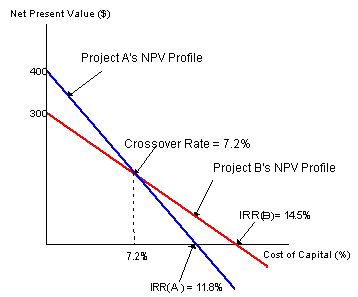# Subject 4. NPV Profiles

A NPV profile is a graph showing the relationship between a project's NPV and the firm's cost of capital. The point where a project's net present value profile crosses the horizontal axis indicates a project's internal rate of return.Some observations:

• The IRR is the discount rate that sets the NPV to 0.
• The NPV profile declines as the discount rate increases.
• Project A has a higher NPV at low discount rates, while Project B has a higher NPV at high discount rates. The NPV profiles of Project A and B join at the crossover rate, at which the projects' NPVs are equal.
• The slope of Project A's NPV profile is steeper. This indicates that Project A's NPV is more sensitive to changes in the discount rates.